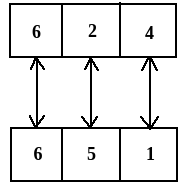# Three different positions of a dice are shown below. How many dots lie opposite 2 dots?Option 1)   4   Option 2)   3   Option 3)   5   Option 4)   6   Option 5)   1

In the above three diagrams,

Neither it is standard dice nor we are observing two common faces in two diagrams.

So, here, in this case,

To find out the opposite faces we will wright all the elements in the clockwise direction starting with the common element in any two figure.

So taking figure (i) & figure (ii) for the above experiment,So the number opposite to number 2 dots = 5 dots

Exams
Articles
Questions